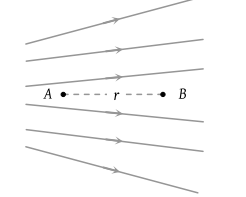• Subject:

...

• Topic:

...

Figure shows the electric lines of force emerging from a charged body. If the electric field at A and B are EA and EB respectively and if the displacement between A and B is r then(1) EA > EB

(2) EA < EB

(3) ${E}_{A}=\frac{{E}_{B}}{r}$

(4) ${E}_{A}=\frac{{E}_{B}}{{r}^{2}}$

PMT